# A Turing-Framework for Evolutionary Processes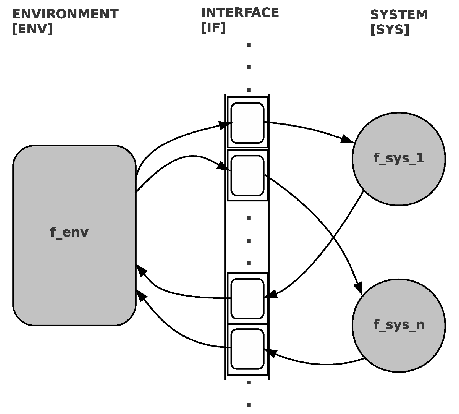To implement an evolutionary process with computers we will use as principal guideline the concept of computability as it has been defined by A.M.Turing  with his concept of a 'Turing Machine' [TM]. Until today this is the most general concept of a computational process and nobody could find a formalism, which demonstrates 'computability' and which is 'stronger' than the TM.

The figure 2.8 shows a first sketch of such a computational framework for evolutionary processes.

• Interface [IF]: The tape of the TM plays the role of an interface between the acting systems and the environment. The environment as well as each system can read ('input') from the interface and they can write ('output') on it.
• Environment [ENV]: The environment is the main actor in an evolutionary process. The environment 'decides' which system is a 'good' system because it is the environment which gives the conditions for 'survival'. Systems which can not cope with the environmental conditions will 'die out'. Therefore the environment will be a TM which simulates conditions and responds to each action (output, write) from the other systems with appropriate 'effects' written as outputs into the interface, which serves as inputs for the systems.
• Systems [SYS]: All actors in the environment, which are needed for an evolutionary process are here assumed as input-output systems, short 'system' [SYS]. In the following experiments this are mainly 'software programs', 'human persons' interacting via environment with these systems, or 'robots' in the real world.
• Time [T]: an important factor in such a simulation (not shown in the diagram) is 'time' [T]. In the light of quantum physics is reality 'partitioned' into 'quanta' which assemble to larger patterns of quanta, etc. In a simulation with TMs time is always assumed to be 'discrete' represented by some technical device called 'clock' [CLK]. Every clock has a minimal 'time unit' which represents the 'precision' with regard to time.
• Interaction: The interaction of inputs and outputs has to follow a certain 'protocol' [PROT] which gives explicit rules which participant can read/ write certain parts of the interface at what time. Such a timing-protocol defines the 'shape' of the possible 'reality' in the simulation.

One way to write this in formulas could be as follows: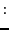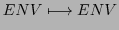(2.5)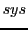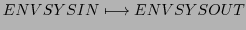(2.6)

In this model(2.5) the environment is assumed as a set of possible 'facts' given as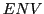. These facts can be changed by the environment function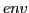. The individual systems within this environment usually can only use a subsetfrom all these environmental states and they produce states which are only subsets of these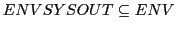(and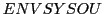have to be written on a tape).

But this simple model(2.5) is lacking the main property which characterizes the dynamics of our world as well as the dynamics of biological life. The functionsandin this model are fixed. From history we know that the functionhas changed all the time, and - depending from the point of view of modeling - as well the function. The other point is, that the start ('birth') and the end ('death') of a biological system - in this case the 'existence' of the function! - is managed by the environmenttoo.

To allow the start and end of a system functionby an environment functionwe have to assume that the system function is given as a description on the tape of the environment. That means a system is from the point of view of the environment a 'fact' which can be manipulated. In technical terms we would say that the environment functionsimulates the system function. Thus we wouldget:(2.7)(2.8)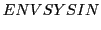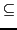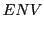(2.9)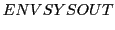(2.10)(2.11)

This model(2.7) tells you that the 'laws' of the environment regulate the appearance of a system. In the next step it opens the possibility that the environment is also responsible for possible changes of the system function. Like in the real world where the 'matter' consisting of atoms and molecules behaving according the laws of chemistry (based on physics) and within this 'field of laws' are 'directed' into 'typical' patterns of molecules' shaping self-reproducing units, we have here the environment functiondetermining how a system function shall be 'look alike' and 'when' it will start and end their 'work'.

This model is still incomplete because we know that the environment functionitself does change because the structure of the world is changing according to 'general laws' and according to the availability of different kinds of 'matter' under the 'circumstances' of a certain area of the universe. In this perspective the environment functionitself has to be assumed as a 'fact' with regard to a 'meta function' which we call here universewhich is operating on 'facts' called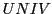. Extending our previous model we have the following formulas: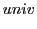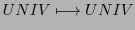(2.12)(2.13)(2.14)(2.15)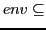(2.16)(2.17)(2.18)(2.19)

Thus, from the point of computability we have different levels of computation where functions of a lower level are 'facts' ('data') for functions of a higher level (cf. figure 2.9). The functions of the higher level always are simulating the functions of a lower level which are - seen from the higher level - 'data'. This schema can be extended infinitely often. But, clearly, it becomes more and more complex and the 'amount' of the 'computational work' is growing2.11.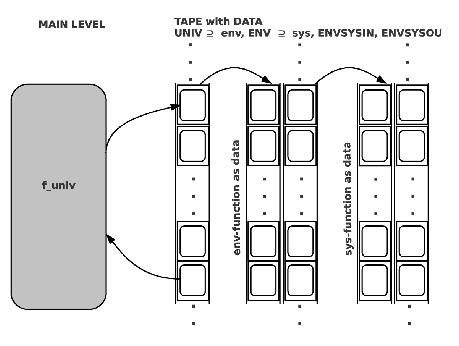For the following engineering process it follows that we have always to be clear to which extend we want to 'model' systems within environments. If we want more and more 'dynamics' in the model we have to model these dynamics with the aid of higher-level functions manipulating the dynamics.

Remarks: One should explain a bit more, whether those kinds of problems, which one wants to solve, are related either to those problems, which are 'computable' or not. The fact, that there exist mathematical concepts which can not be 'computed' alerts for the possibility, that some problems cannot be 'computed'. But because many mathematical problems have no counterpart in reality this 'being not computable' must therefore not inevitably be a problem for the problem at hand.

The other point is that to recognize a problem as 'not being computable' must also not be a counterargument against the use of a turing machine as benchmark. The turing machine can still compute 'non-computable problems', but there will eventually be no solution. But in this case no other device can do either. Besides this it is often the case that most 'non-computable' problems can be analyzed as 'hierarchies of functions' where every single function of the hierarchy can be computed, but the whole hierarchy as such not. To give up the concept of a turing machine would destroy any possibility to continue talking about any kind of computation.

Gerd Doeben-Henisch 2013-01-14